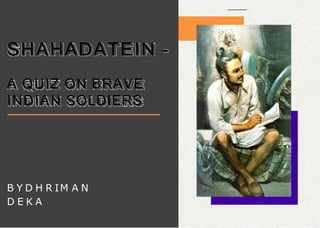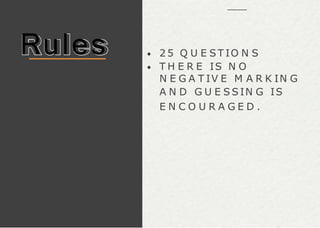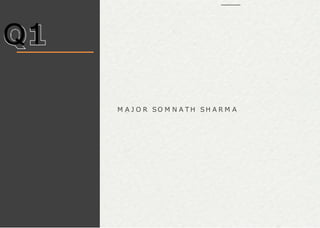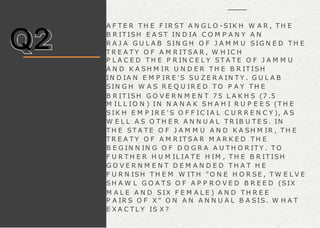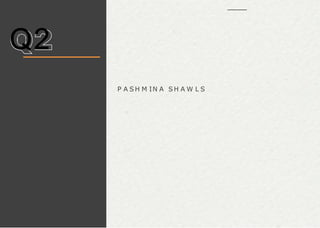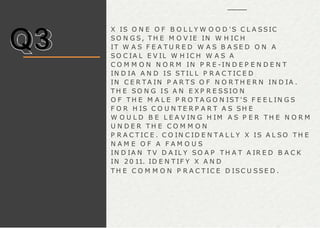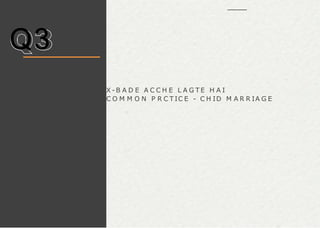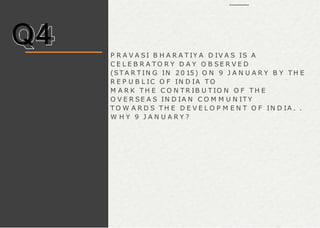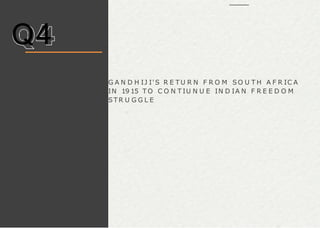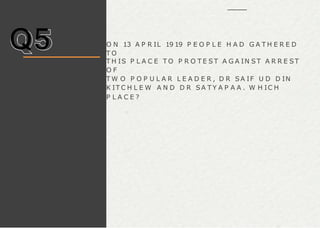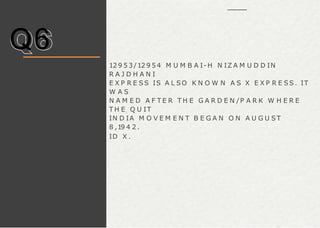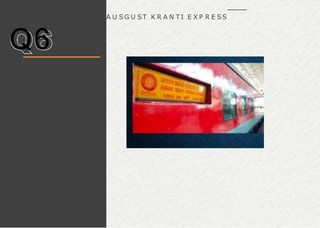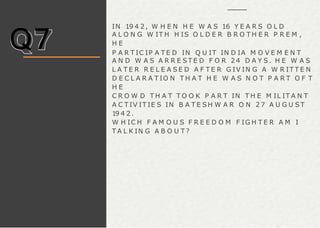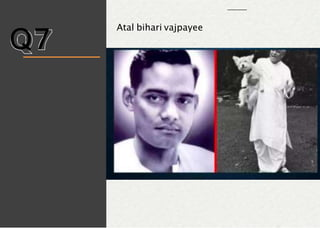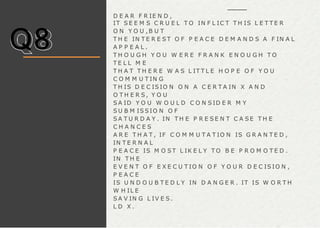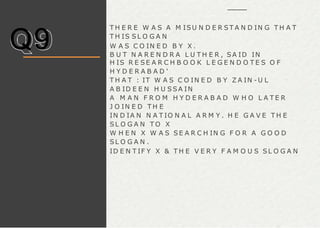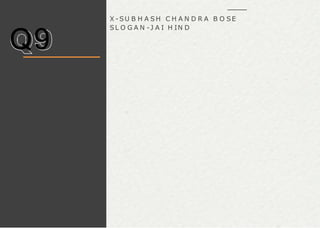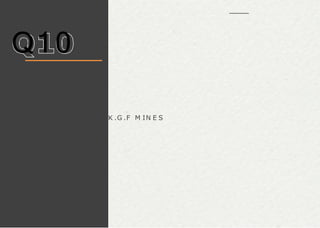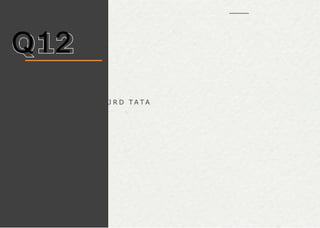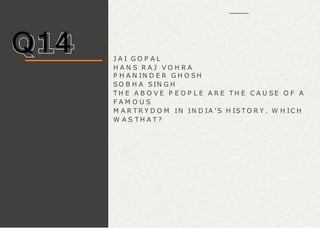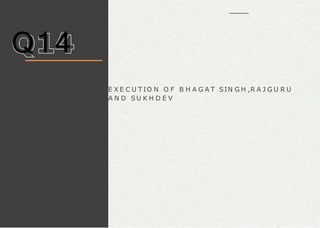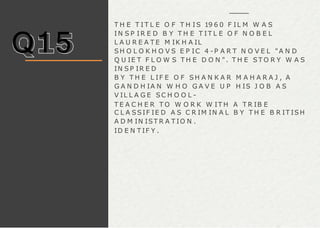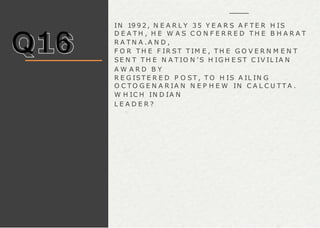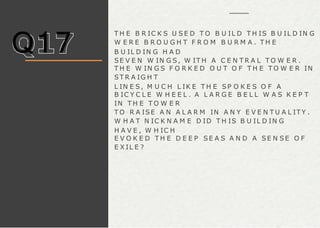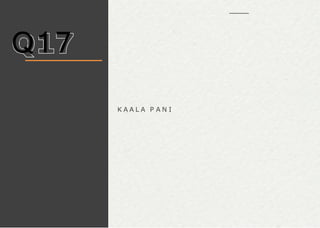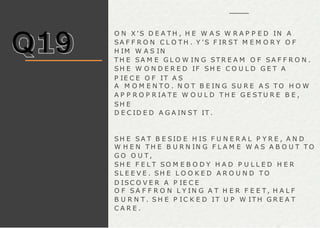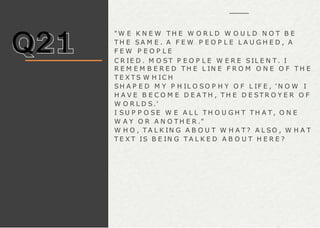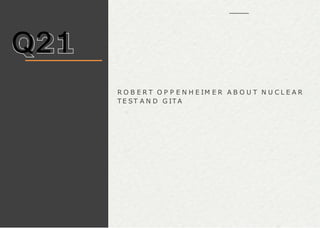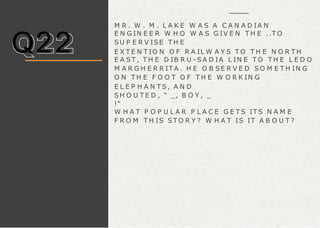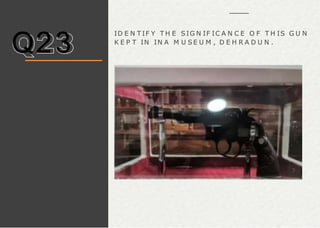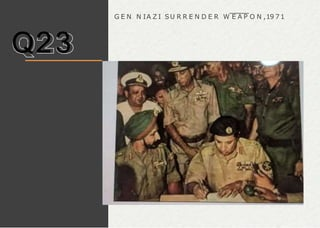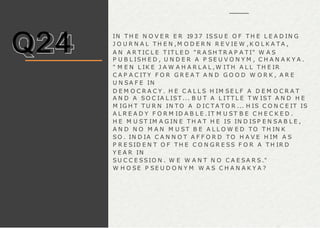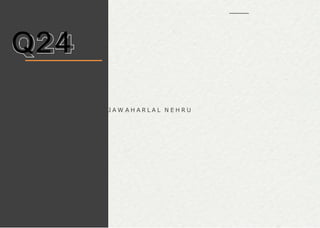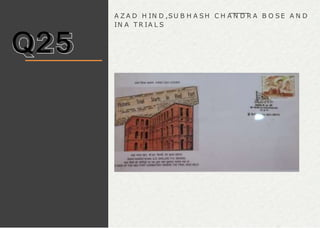1 de 54

1. SHAHADATEIN - A QUIZ ON BRAVE INDIAN SOLDIERS B Y D H R IM A N D E K A
2. Rules 2 5 Q U E ST IO N S T H E R E IS N O N E G A T IV E M A R K IN G A N D G U E S S IN G IS E N C O U R A G E D .
3. Quiz starts from following slide.
4. Q1 X A T T H E Y O U N G A G E O F 2 5 Y E A R S L A ID D O W N H IS L IF E F O R T H E N A T IO N A N D B E C A M E T H E R E C IP IE N T O F F IR S T “ P A R A M V IR C H A K R A ” O F IN D E P E N D E N T IN D IA . F IG H T IN G IN T H E IN D O - P A K IS T A N I W A R O F 19 4 7 - 19 4 8 , H E W A S M A R T Y R E D IN A C T IO N O N 3 N O V E M B E R 19 4 7 W H IL E R E P U L SIN G P A K IST A N I IN F IL T R A T O R S N E A R S R IN A G A R A IR P O R T . H IS L A ST M E SSA G E T O B R IG A D E H Q R E C E IV E D A F E W M O M E N T S B E F O R E H E W A S K IL L E D W A S : “ T H E E N E M Y IS O N L Y 5 0 Y A R D S F R O M U S . W E A R E H E A V IL Y O U T N U M B E R E D . W E A R E U N D E R D E V A ST A T IN G F IR E . I SH A L L N O T W IT H D R A W A N IN C H B U T W IL L F IG H T T O T H E L A ST M A N A N D T H E L A ST R O U N D .” ID E N T IF Y X .
5. Q1 M A J O R SO M N A T H SH A R M A
6. Q2 A F T E R T H E F IR ST A N G L O -SIK H W A R , T H E B R IT ISH E A ST IN D IA C O M P A N Y A N R A J A G U L A B SIN G H O F J A M M U SIG N E D T H E T R E A T Y O F A M R IT S A R , W H IC H P L A C E D T H E P R IN C E L Y S T A T E O F J A M M U A N D K A SH M IR U N D E R T H E B R IT ISH IN D IA N E M P IR E 'S SU Z E R A IN T Y . G U L A B SIN G H W A S R E Q U IR E D T O P A Y T H E B R IT ISH G O V E R N M E N T 7 5 L A K H S (7 .5 M IL L IO N ) IN N A N A K S H A H I R U P E E S (T H E S IK H E M P IR E ' S O F F IC IA L C U R R E N C Y ), A S W E L L A S O T H E R A N N U A L T R IB U T E S. IN T H E ST A T E O F J A M M U A N D K A SH M IR , T H E T R E A T Y O F A M R IT S A R M A R K E D T H E B E G IN N IN G O F D O G R A A U T H O R IT Y . T O F U R T H E R H U M IL IA T E H IM , T H E B R IT ISH G O V E R N M E N T D E M A N D E D T H A T H E F U R N ISH T H E M W IT H "O N E H O R SE , T W E L V E SH A W L G O A T S O F A P P R O V E D B R E E D (SIX M A L E A N D SIX F E M A L E ) A N D T H R E E P A IR S O F X " O N A N A N N U A L B A SIS. W H A T E X A C T L Y IS X ?
7. Q2 P A SH M IN A SH A W L S
8. Q3 X IS O N E O F B O L L Y W O O D ' S C L A SSIC SO N G S, T H E M O V IE IN W H IC H IT W A S F E A T U R E D W A S B A S E D O N A SO C IA L E V IL W H IC H W A S A C O M M O N N O R M IN P R E -IN D E P E N D E N T IN D IA A N D IS ST IL L P R A C T IC E D IN C E R T A IN P A R T S O F N O R T H E R N IN D IA . T H E SO N G IS A N E X P R E SSIO N O F T H E M A L E P R O T A G O N IST 'S F E E L IN G S F O R H IS C O U N T E R P A R T A S SH E W O U L D B E L E A V IN G H IM A S P E R T H E N O R M U N D E R T H E C O M M O N P R A C T IC E . C O IN C ID E N T A L L Y X IS A L SO T H E N A M E O F A F A M O U S IN D IA N T V D A IL Y SO A P T H A T A IR E D B A C K IN 2 0 11. ID E N T IF Y X A N D T H E C O M M O N P R A C T IC E D ISC U SS E D .
9. Q3 X - B A D E A C C H E L A G T E H A I C O M M O N P R C T IC E - C H ID M A R R IA G E
10. Q4 P R A V A SI B H A R A T IY A D IV A S IS A C E L E B R A T O R Y D A Y O B SE R V E D (ST A R T IN G IN 2 0 15 ) O N 9 J A N U A R Y B Y T H E R E P U B L IC O F IN D IA T O M A R K T H E C O N T R IB U T IO N O F T H E O V E R SE A S IN D IA N C O M M U N IT Y T O W A R D S T H E D E V E L O P M E N T O F IN D IA . . W H Y 9 J A N U A R Y ?
11. Q4 G A N D H IJ I'S R E T U R N F R O M SO U T H A F R IC A IN 19 15 T O C O N T IU N U E IN D IA N F R E E D O M ST R U G G L E
12. Q5 O N 13 A P R IL 19 19 P E O P L E H A D G A T H E R E D T O T H IS P L A C E T O P R O T E S T A G A IN ST A R R E ST O F T W O P O P U L A R L E A D E R , D R SA IF U D D IN K IT C H L E W A N D D R SA T Y A P A A . W H IC H P L A C E ?
13. Q5 J A L IA N W A L A H B A G H
14. Q6 12 9 5 3 /12 9 5 4 M U M B A I- H N IZ A M U D D IN R A J D H A N I E X P R E SS IS A L SO K N O W N A S X E X P R E SS . IT W A S N A M E D A F T E R T H E G A R D E N /P A R K W H E R E T H E Q U IT IN D IA M O V E M E N T B E G A N O N A U G U ST 8 ,19 4 2 . ID X .
15. Q6 A U SG U ST K R A N T I E X P R E SS
16. Q7 IN 19 4 2 , W H E N H E W A S 16 Y E A R S O L D A L O N G W IT H H IS O L D E R B R O T H E R P R E M , H E P A R T IC IP A T E D IN Q U IT IN D IA M O V E M E N T A N D W A S A R R E ST E D F O R 2 4 D A Y S. H E W A S L A T E R R E L E A S E D A F T E R G IV IN G A W R IT T E N D E C L A R A T IO N T H A T H E W A S N O T P A R T O F T H E C R O W D T H A T T O O K P A R T IN T H E M IL IT A N T A C T IV IT IE S IN B A T E SH W A R O N 2 7 A U G U ST 19 4 2 . W H IC H F A M O U S F R E E D O M F IG H T E R A M I T A L K IN G A B O U T ?
17. Q7 Atal bihari vajpayee
18. Q8 D E A R F R IE N D , IT S E E M S C R U E L T O IN F L IC T T H IS L E T T E R O N Y O U , B U T T H E IN T E R E S T O F P E A C E D E M A N D S A F IN A L A P P E A L . T H O U G H Y O U W E R E F R A N K E N O U G H T O T E L L M E T H A T T H E R E W A S L IT T L E H O P E O F Y O U C O M M U T IN G T H IS D E C IS IO N O N A C E R T A IN X A N D O T H E R S , Y O U S A ID Y O U W O U L D C O N S ID E R M Y S U B M IS S IO N O F S A T U R D A Y . IN T H E P R E S E N T C A S E T H E C H A N C E S A R E T H A T , IF C O M M U T A T IO N IS G R A N T E D , IN T E R N A L P E A C E IS M O S T L IK E L Y T O B E P R O M O T E D . IN T H E E V E N T O F E X E C U T IO N O F Y O U R D E C IS IO N , P E A C E IS U N D O U B T E D L Y IN D A N G E R . IT IS W O R T H W H IL E S A V IN G L IV E S . L D X .
19. Q8 E X E C U T IO N O F
20. Q9 T H E R E W A S A M ISU N D E R ST A N D IN G T H A T T H IS S L O G A N W A S C O IN E D B Y X . B U T N A R E N D R A L U T H E R , SA ID IN H IS R E SE A R C H B O O K L E G E N D O T E S O F H Y D E R A B A D ' T H A T : IT W A S C O IN E D B Y Z A IN -U L A B ID E E N H U SSA IN A M A N F R O M H Y D E R A B A D W H O L A T E R J O IN E D T H E IN D IA N N A T IO N A L A R M Y . H E G A V E T H E SL O G A N T O X W H E N X W A S SE A R C H IN G F O R A G O O D SL O G A N . ID E N T IF Y X & T H E V E R Y F A M O U S SL O G A N
21. Q9 X - SU B H A SH C H A N D R A B O SE SL O G A N -J A I H IN D
22. Q10 • 2 N D D E E P E ST M IN E IN T H E W O R L D . •B R IT ISH E R S F O N D L Y C A L L E D M IN I E N G L A N D . • A N D C IT Y T O B E E L E C T R IF IE D IN A S IA . •1ST H Y D R O E L E C T R IC S T A T IO N IN A S IA , SH IV A SA M U D R A P R O V ID IN G E L E C T R IC IT Y H E R E . •IT S N A M E IS SA M E A S T H E N A M E O F A 2 0 18 B L O C K B U ST E R M O V IE . ID T H E C IT Y ?
23. Q10 K .G .F M IN E S
24. Q11 X W A S A N IN D U ST R IA L IST , P H IL A N T H R O P IS T & A N IN D IA N F R E E D O M F IG H T E R . H E F O U N D E D Y G R O U P O F C O M P A N IE S IN T H E 19 2 0 S & T H E G R O U P N O W H A S 2 4 C O M P A N IE S. H E W A S A L SO A C L O SE A N D B E L O V E D A SSO C IA T E O F M A H A T M A G A N D H I, W H O IS K N O W N T O O F T E N D E C L A R E T H A T X W A S H IS 5 T H S O N . H E W A S A L SO D U B B E D A S T H E 'M E R C H A N T O F T H E P R IN C E ' B Y G A N D H I
25. Q11 X - J A M N A L A L B A J A J Y -B A J A J
26. Q12 G A N G A D H A R T IL A K A L O N G W IT H M U M M O H A N D A A R A M J I & IN D U S T R IA L IST X C O N C IE V E D T H E B O M B A Y SW A D E SH I C O -O P ST O R E S C O . L T D . IN F A V O U R O F SW A D E SH I G O O D S & B O Y C O T T IN G F O R E IG N G O O D S IN E A R L Y 19 0 0 S. T H E ST O R E IS K N O W N T O D A Y A S B O M B A Y ST O R E . ID X
27. Q12 J R D T A T A
28. Q13 M IR T A J M U H A M M E D K H A N W A S A IN D IA N F R E E D O M F IG H T E R W H O B E C A M E A P A R T O F F R O N T IE R G A N D H I K H A N A B D U L G A F F A R K H A N 'S M O V E M E N T & H A D E E V E N C O N T E ST E D E L E C T IO N A G A IN ST A N O T H E R N A T IO N A L IC O N , M A U L A N A A B D U L K A L A M A Z A D & L O S T . H E W A S A L SO A P A R T O F Q U IT IN D IA M O V E M E N T , W H IL E H IS F A T H E R -IN - L A W SE R V E D IN M A N G A L O R E A S T H E C H IE F E N G IN E E R O F T H E P O R T IN 19 6 0 . B U T T H E IR SO N /G R A N D SO N IS F A M O U S IN & E N T IR E L Y D IF F E R E N T F IE L D . L D T H IS P E R SO N A L IT Y
29. Q13 SH A H R U K H K H A N
30. Q14 J A I G O P A L H A N S R A J V O H R A P H A N IN D E R G H O SH SO B H A SIN G H T H E A B O V E P E O P L E A R E T H E C A U SE O F A F A M O U S M A R T R Y D O M IN IN D IA ' S H IS T O R Y . W H IC H W A S T H A T ?
31. Q14 E X E C U T IO N O F B H A G A T SIN G H ,R A J G U R U A N D SU K H D E V
32. Q15 T H E T I T L E O F T H I S 19 6 0 F IL M W A S IN S P IR E D B Y T H E T IT L E O F N O B E L L A U R E A T E M IK H A IL SH O L O K H O V S E P IC 4 -P A R T N O V E L "A N D Q U IE T F L O W S T H E D O N ". T H E ST O R Y W A S IN S P IR E D B Y T H E L IF E O F SH A N K A R M A H A R A J , A G A N D H IA N W H O G A V E U P H IS J O B A S V IL L A G E SC H O O L - T E A C H E R T O W O R K W IT H A T R IB E C L A SSIF IE D A S C R IM IN A L B Y T H E B R IT ISH A D M IN IST R A T IO N . ID E N T IF Y .
33. Q15 T H E T I T L E O F T H I S 19 6 0 F IL M W A S IN S P IR E D B Y T H E T IT L E O F N O B E L L A U R E A T E M IK H A IL SH O L O K H O V S E P IC 4 -P A R T N O V E L "A N D Q U IE T F L O W S T H E D O N ". T H E ST O R Y W A S IN S P IR E D B Y T H E L IF E O F SH A N K A R M A H A R A J , A G A N D H IA N W H O G A V E U P H IS J O B A S V IL L A G E SC H O O L - T E A C H E R T O W O R K W IT H A T R IB E C L A SSIF IE D A S C R IM IN A L B Y T H E B R IT ISH A D M IN IST R A T IO N . ID E N T IF Y .
34. Q15 J IS D E SH M E IN G A N G A B E H T I H A I
35. Q16 IN 19 9 2 , N E A R L Y 3 5 Y E A R S A F T E R H IS D E A T H , H E W A S C O N F E R R E D T H E B H A R A T R A T N A .A N D , F O R T H E F IR ST T IM E , T H E G O V E R N M E N T SE N T T H E N A T IO N 'S H IG H E ST C IV IL IA N A W A R D B Y R E G IST E R E D P O ST , T O H IS A IL IN G O C T O G E N A R IA N N E P H E W IN C A L C U T T A . W H IC H IN D IA N L E A D E R ?
36. Q16 M A U L A N A A B D U L K A L A M A Z A D
37. Q17 T H E B R IC K S U S E D T O B U IL D T H IS B U IL D IN G W E R E B R O U G H T F R O M B U R M A . T H E B U IL D IN G H A D SE V E N W IN G S , W IT H A C E N T R A L T O W E R . T H E W IN G S F O R K E D O U T O F T H E T O W E R IN ST R A IG H T L IN E S , M U C H L IK E T H E SP O K E S O F A B IC Y C L E W H E E L . A L A R G E B E L L W A S K E P T IN T H E T O W E R T O R A ISE A N A L A R M IN A N Y E V E N T U A L IT Y . W H A T N IC K N A M E D ID T H IS B U IL D IN G H A V E , W H IC H E V O K E D T H E D E E P SE A S A N D A SE N S E O F E X IL E ?
38. Q17 K A A L A P A N I
39. Q18 N A M E T H E SO N O F A D IW A N O F A SM A L L P R IN C E L Y ST A T E IN T H E K A T H IA W A R R E G IO N A N D H IS F O U R T H W IF E ?
40. Q18 M K G A N D H I
41. Q19 O N X 'S D E A T H , H E W A S W R A P P E D IN A SA F F R O N C L O T H . Y ' S F IR ST M E M O R Y O F H IM W A S IN T H E SA M E G L O W IN G ST R E A M O F S A F F R O N . SH E W O N D E R E D IF SH E C O U L D G E T A P IE C E O F IT A S A M O M E N T O . N O T B E IN G S U R E A S T O H O W A P P R O P R IA T E W O U L D T H E G E ST U R E B E , SH E D E C ID E D A G A IN ST IT . SH E S A T B E SID E H IS F U N E R A L P Y R E , A N D W H E N T H E B U R N IN G F L A M E W A S A B O U T T O G O O U T , SH E F E L T SO M E B O D Y H A D P U L L E D H E R SL E E V E . SH E L O O K E D A R O U N D T O D ISC O V E R A P IE C E O F S A F F R O N L Y IN G A T H E R F E E T , H A L F B U R N T . S H E P IC K E D IT U P W IT H G R E A T C A R E .
42. Q19 Y -SIST E R N IV E D IT A X - SW Q A M I V IV E K A N A N D
43. Q20 F O R E SE E IN G T H E P O T E N T IA L U P C O M IN G D A N G E R S, X P L A N N E D T O B U Y T H E G O A P O R T F R O M T H E P O R T U G U E SE T O E N S U R E A N E SC A P E R O U T E . H E C A M E V E R Y C L O S E T O A C T U A L L Y D O IN G IT W IT H T H E H E L P O F B R IT IS H M ID D L E M E N , B E F O R E T H E D E A L F E L L O F F D U E T O O T H E R M A J O R ISS U E S. F O L L O W IN G IS A N E X C E R P T O F T H E A G R E E M E N T : "T H E G O V E R N M E N T O F T H E P O R T U G U E SE R E P U B L IC A N D B E IN G D E SIR O U S O F R E G U L A T IN G T R A F F IC IN T H E P O R T A N D R A IL W A Y O F M A R M A G O A IN P O R T U G U E S E IN D IA ,A N D T E R R IT O R IE S H A V E R E S O L V E D T O E N T E R IN T O A C O N V E N T IO N F O R T H A T P U R P O S E ."
44. Q20 M IR O SM A N A L I,N IZ A M O F H Y D E R A B A D
45. Q21 "W E K N E W T H E W O R L D W O U L D N O T B E T H E SA M E . A F E W P E O P L E L A U G H E D , A F E W P E O P L E C R IE D . M O ST P E O P L E W E R E S IL E N T . I R E M E M B E R E D T H E L IN E F R O M O N E O F T H E T E X T S W H IC H SH A P E D M Y P H IL O SO P H Y O F L IF E , ' N O W I H A V E B E C O M E D E A T H , T H E D E ST R O Y E R O F W O R L D S.' I S U P P O SE W E A L L T H O U G H T T H A T , O N E W A Y O R A N O T H E R ." W H O , T A L K IN G A B O U T W H A T ? A L SO , W H A T T E X T IS B E IN G T A L K E D A B O U T H E R E ?
46. Q21 R O B E R T O P P E N H E IM E R A B O U T N U C L E A R T E ST A N D G IT A
47. Q22 M R . W . M . L A K E W A S A C A N A D IA N E N G IN E E R W H O W A S G IV E N T H E ..T O SU P E R V ISE T H E E X T E N T IO N O F R A IL W A Y S T O T H E N O R T H E A ST , T H E D IB R U -S A D IA L IN E T O T H E L E D O M A R G H E R R IT A . H E O B SE R V E D SO M E T H IN G O N T H E F O O T O F T H E W O R K IN G E L E P H A N T S , A N D SH O U T E D , " _, B O Y , _ !" W H A T P O P U L A R P L A C E G E T S IT S N A M E F R O M T H IS ST O R Y ? W H A T IS IT A B O U T ?
48. Q22 D IG B O I
49. Q23 ID E N T IF Y T H E SIG N IF IC A N C E O F T H IS G U N K E P T IN IN A M U S E U M , D E H R A D U N .
50. Q23 G E N N IA Z I SU R R E N D E R W E A P O N ,19 7 1
51. Q24 IN T H E N O V E R E R 19 37 ISSU E O F T H E L E A D IN G J O U R N A L T H E N ,M O D E R N R E V IE W ,K O L K A T A , A N A R T IC L E T IT L E D "R A SH T R A P A T I" W A S P U B L ISH E D , U N D E R A P SE U V O N Y M , C H A N A K Y A . " M E N L IK E J A W A H A R L A L ,W IT H A L L T H E IR C A P A C IT Y F O R G R E A T A N D G O O D W O R K , A R E U N SA F E IN D E M O C R A C Y . H E C A L L S H IM SE L F A D E M O C R A T A N D A SO C IA L IST ... B U T A L IT T L E T W IST A N D H E M IG H T T U R N IN T O A D IC T A T O R ... H IS C O N C E IT IS A L R E A D Y F O R M ID A B L E .IT M U ST B E C H E C K E D . H E M U ST IM A G IN E T H A T H E IS IN D IS P E N SA B L E , A N D N O M A N M U ST B E A L L O W E D T O T H IN K SO . IN D IA C A N N O T A F F O R D T O H A V E H IM A S P R E SID E N T O F T H E C O N G R E SS F O R A T H IR D Y E A R IN SU C C E SSIO N . W E W A N T N O C A E SA R S." W H O SE P SE U D O N Y M W A S C H A N A K Y A ?
52. Q24 J A W A H A R L A L N E H R U
53. Q25 T H E SE C A R D S, SIG N E D B Y SO M E 250 ,0 0 0 IN D IA N S SW E A R IN G T H E IR A L L E G IA N C E TO X , W E R E A F IR ST -O F -IT S-K IN D SO C IA L E X P E R IM E N T B Y X 'S L E A D E R Y , A C C O R D IN G TO W H O M , P E O P L E O F IN D IA M A T TE R E D M O R E T H A N T H E L A N D . SO M E Y E A R S L A TE R , IN W H A T H IST O R IC A L E V E N T IN V O L V IN G SO M E O B IG G E ST L A W Y E R S IN IN D IA L IK E J .L . N E H R U , A SA F A L I A N D K .M .K A T J U , W E R E TH E SE C A R D S U SE D A S A N E V ID E N C E A G A IN ST TH E "O F F E N D E R S"? F O R F U L L P O IN T S, X , Y A N D TH E E V E N T .
54. Q25 A Z A D H IN D ,SU B H A SH C H A N D R A B O SE A N D IN A T R IA L S